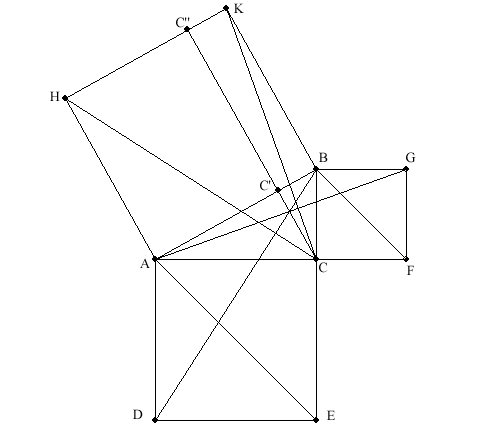# THE PROOF OF PYTHAGOREAN THEOREM

Pythagoras Theorem
If ABC is a triangle and <)ACB is the right angle then |AC|^2 + |BC|^2 = |AB|^2.

Proof
Take any triangle ABC with <)ACB right.
Let DECA, CFGB, ABKH be squares.
Let C'C be the altitude of triangle ACB. And let <)C''C'B be right.

We want to show that |AC|^2 + |BC|^2 = |AB|^2.
The area of a square is the second power of the length of its side. Then it is enough to show that the sum of the areas of DECA and CFGB is equal to the area of ABKH.
Hence it is enough to show that the sum of the areas of triangles DEA and FGB is equal to half of the area of ABKH.DE is the altitude of triangle DEA.
Since <)ACB is right, segments AD and EB are parallel. Hence triangels DBA and DEA have the same length of altitudes and the same base.
So the area of triangle DBA is equal to the area of triangle DEA.

Triangles DBA and CHA are congruent because |AD|=|AC| and |AB|=|AH| and
<)DAB = 90 degrees + <)CAB = <)CAH.
Hence the area of triangle CHA is equal to the area of triangle DBA.

Since C'C is the altitude of triangle ACB and <)C''C'B is right, segments AH and CC'' are parallel. Hence the area of triangle CHA is (1/2)*|AH|*|HC''|. And this is equal to half of the area of rectangle AC'C''H.

We have shown that the area of triangle DEA is (1/2)*|AH|*|HC''|.

Now we will show that the area of triangle FGB is (1/2)*|BK|*|C''K|.

FG is the altitude of triangle FGB.
Since <)ACB is right, segments BG and AF are parallel. Hence triangels FGB and AGB have the same length of altitudes and the same base.
So the area of triangle FGB is equal to the area of triangle AGB.

Triangles AGB and KCB are congruent because |BG|=|BC| and |AB|=|BK| and
<)ABG = 90 degrees + <)CBA = <)CBK.
Hence the area of triangle KCB is equal to the area of triangle AGB.

Since C'C is the altitude of triangle ACB and <)C''C'B is right, segments BK and CC'' are parallel. Hence the area of triangle KCB is (1/2)*|BK|*|C''K|. And this is equal to half of the area of rectangle C'BKC''.

We have shown that the area of triangle FGB is (1/2)*|BK|*|C''K|.
Earlier we showed that the area of triangle DEA is (1/2)*|AH|*|HC''|.

(1/2)*|AH|*|HC''| + (1/2)*|BK|*|C''K| = (1/2)*|AH|*|HK|.
And this shows that the sum of the areas of triangles DEA and FGB is equal to half of the area of ABKH.
Now the proof is complete.